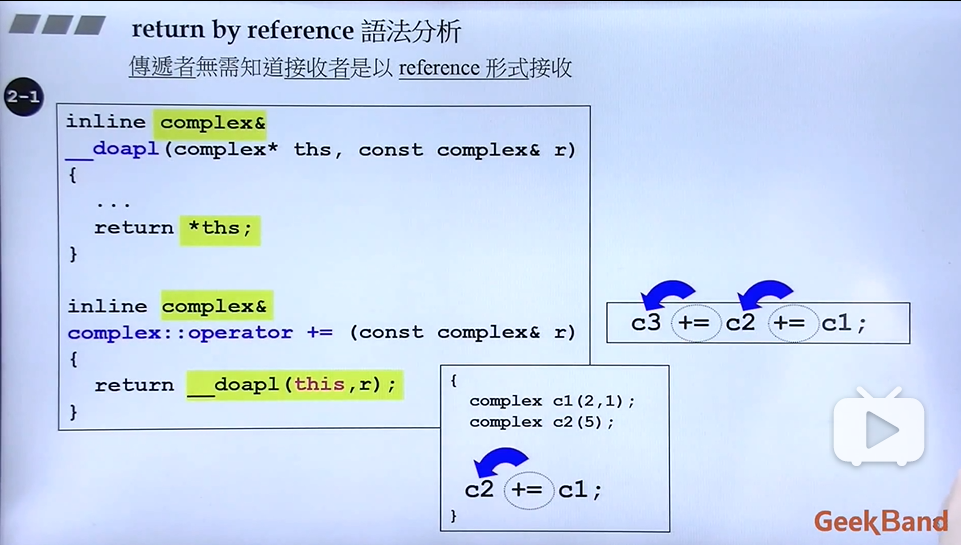# 从复数类实现中看到精髓--面向对象高级开发学习系列

## 声明

### inline和宏

inline和宏差不多,方法直接写在定义的地方是inline

inline取决于编译器实现

public放给外界使用的接口

### 构造函数

• 可以默认值,当然其他函数也可以这样
• 一般使用complex(double r=0,double i=0):re(r),im(i){}初始化列 里面写，这样写得很大气(在初始化列初始化)
• 构建赋值由声明顺序决定
• 没有返回类型—不过曾经学jni发现是返回类对象的指针
• 可以有多个构造函数,可以重载(常用于构造函数),编译器会编码成不同的东西

public:
complex(double r = 0, double i = 0)
: re (r), im (i)
{ }
complex () : re(0), im(0) { }

{
// 下面会导致ambiguous
complex a;
complex b();
}

• singleton单例设计模式把构造函数写在private(老师说暂时不深入)

### const member functions(常量成员函数)

double real () const { return re; }

{
const complex c1(2,1);
// 如果前面方法没有用const,而你定义的c1是const
// 这样你使用下面的输出编译器会报错
// 因为它以为你可能会改变c1中的值,所以不准
cout<< c1.real();
cout<< c1.imag();
}


### pass by value vs. pass by reference

C语言有指针传递

complex& operator += (const complex&);


### 相同的class的各个objects互为友元

class complex{
public:

// 这里没有通过函数拿,而是直接拿数据
int func(const complex& param)
{ return param.re + param.im; }
}


### 老师说检查学生写的类注意点

1. 数据在private
2. 参数尽可能引用,看情况const
3. 返回值尽可能引用传递
4. body本体中应该加const就要加(别人要不要用const都可以了)
5. 初始化赋值尽量使用，尽量不要很晚了在{}内赋值

### 操作符重载1–成员函数_有this

• C++里面操作符就相当于一个函数,而C语言不是
• 写个plus函数不好,所以C++容许你重载+
• 谁调用我，谁就是this(写代码看不到this传参)，任何成员函数都隐藏了this

### return by reference

#### 连串赋值

inline complex&
__doapl(complex* ths, const complex& r)
{
...
return *ths;
}

inline complex&
complex::operator += (const complex& r)
{
return __doapl(this,r);
}

{
complex c1(2,1);
complex c2(5);

c2 += c1;
c3 += c2 += c1;
}### 操作符重载2–非成员函数_无this

// 这里 加 的返回值应该是对象，不能传reference，否则传出去一个指针，然后内存回收掉了，然后就很奇怪了
// c = a + b;
inline complex
operator + (const complex& x, const complex& y)
{
return complex(real(x) + real(y),
imag(x) + imag(y));
}


### 关于直接返回引用的测试代码

#include<bits/stdc++.h>
using namespace std;

// error: invalid initialization of reference of type 'int&' from expression of type 'const int'
//      return a;
//             ^

// 我试了一下，发现是不行的啊
// 等下，a变成了const int?!那应该就是说其实return之后应该也可以
// 变成int吧，难道老师说错了，待会试试复数类的东西吧

// 后面复习的时候突然想到了为什么这里不行了
// 因为这里a 已经变成了一个临时变量了?
// 然后自己尝试把const去掉，编译成功了
// 所以就是说接受者const,传参者随意
// 但是如果接受者不const,传参者不能const
// 这就是之前老师说的你设计的函数尽量加const！
// int & zheng(const int& a)
// {
//     return a;
// }
// 引用真香!!!!!

int & zheng(int& a)
{
return a;
}

const int & zheng3(const int & a)
{
return a;
}

int zheng2(const int& a)
{
return a;
}

int main(){
int a = 233;
cout<< zheng(a)<<endl;
cout<< zheng2(a)<<endl;
cout<< zheng3(a)<<endl;

return 0;
}


## 全部讲解用到代码

#ifndef __COMPLEX__
#define __COMPLEX__

// template<typename T>
class complex
{
public:
complex (double r=0, double i=0)
: re (r) ,im (i)
{ }

complex& operator += (const complex&);
double real () const { return re; }
double imag () const { return im; }
private:
double re, im;

friend complex& __doapl (complex*,
const complex&);

};

inline complex&
__doapl(complex* ths, const complex& r)
{
ths->re += r.re;
ths->im += r.im;
return *ths;
}

inline complex &
complex::operator += (const complex & r)
{
return __doapl (this, r);
}

inline complex
operator + (const complex& x, const complex& y)
{
return complex (real(x) + real(y),
imag(x) + imag(y) );
}

inline complex
operator + (const complex& x, double y)
{
return complex (real(x) + y, imag(x));
}

inline complex
operator + (double x, const complex& y)
{
return complex (x + real(y), imag(y));
}

// 因为不考虑 c1 << cout; 所以无法使用成员函数定义
// 因为成员函数定义要用对象在左边
#include <iostream.h>
ostream&
operator << (ostream& os, const complex& x)
{
return os << '(' << real (x) << ','
<< imag (x) << ')';
}

#endif


©️2019 CSDN 皮肤主题: 技术工厂 设计师: CSDN官方博客